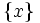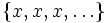# US implies T1

This article gives the statement and possibly, proof, of an implication relation between two topological space properties. That is, it states that every topological space satisfying the first topological space property (i.e., US-space) must also satisfy the second topological space property (i.e., T1 space)
View all topological space property implications | View all topological space property non-implications

## Statement

Any US-space (i.e., a space where every convergent sequence has a unique limit) is a T1 space (i.e., every point is closed).

## Proof

Given: A US-space$X$.

To prove:$X$ is a$T_1$-space.

Proof: We need to show that for every point$x \in X$, the singleton subset$\{ x \}$ is closed. For this, take the sequence$\{ x,x,x, \dots \}$. By the definition of limit,$x$ is a limit of the sequence, and hence, since the space is US, it is the only limit. Thus, for any point$y \ne x$, there exists an open neighborhood of$y$ not containing$x$. Thus,$\{ x \}$ is closed.